Courses

# Intermediate Code Forms Computer Science Engineering (CSE) Notes | EduRev

## Computer Science Engineering (CSE) : Intermediate Code Forms Computer Science Engineering (CSE) Notes | EduRev

The document Intermediate Code Forms Computer Science Engineering (CSE) Notes | EduRev is a part of the Computer Science Engineering (CSE) Course Compiler Design.
All you need of Computer Science Engineering (CSE) at this link: Computer Science Engineering (CSE)

1. Intermediate code forms:

An intermediate code form of source program is an internal form of a program created by the compiler while translating the program created by the compiler while translating the program from a high –level language to assembly code(or)object code(machine code).an intermediate source form represents a more attractive form of target code than does assembly. An optimizing Compiler performs optimizations on the intermediate source form and produces an object module.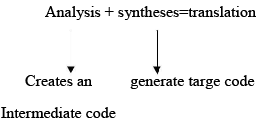In the analysis –synthesis model of a compiler, the front-end translates a source program into an intermediate representation from which the back-end generates target code, in many compilers the source code is translated into a language which is intermediate in complexity between a HLL and machine code .the usual intermediate code introduces symbols to stand for various temporary quantities.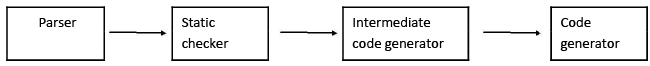position of intermediate code generator

We assume that the source program has already been parsed and statically checked.. the various intermediate code forms are:

a) Polish notation
b) Abstract syntax trees(or)syntax trees
e) Indirect triples
f) Abstract machine code(or)pseudocopde a. postfix notation:

The ordinary (infix) way of writing the sum of a and b is with the operator in the middle: a+b. the postfix (or postfix polish)notation for the same expression places the operator at the right end, as ab+.
In general, if e1 and e2 are any postfix expressions, and Ø to the values denoted by e1 and e2 is indicated in postfix notation nby e1e2Ø.no parentheses are needed in postfix notation because the position and priority (number of arguments) of the operators permits only one way to decode a postfix expression.

Example:

1. (a+b)*c in postfix notation is ab+c*,since ab+ represents the infix expression(a+b).

2. a*(b+c)is abc+* in postfix.

3. (a+b)*(c+d) is ab+cd+* in postfix.
Postfix notation can be generalized to k-ary operators for any k>=1.if k-ary operator Ø is applied to postfix expression e1,e2,……….ek, then the result is denoted by e1e2…….ek Ø. if we know the priority of each operator then we can uniquely decipher any postfix expression by scanning it from either end.

Example:

Consider the postfix string ab+c*.

The right hand * says that there are two arguments to its left. since the next –to-rightmost symbol is c, simple operand, we know c must be the second operand of *.continuing to the left, we encounter the operator +.we know the sub expression ending in + makes up the first operand of *.continuing in this way ,we deduce that ab+c* is “parsed” as (((a,b)+),c)*.

b.  syntax tree:

The parse tree itself is a useful intermediate-language representation for a source program, especially in optimizing compilers where the intermediate code needs to extensively restructure.

A parse tree, however, often contains redundant information which can be eliminated, Thus producing a more economical representation of the source program. One such variant of a parse tree is what is called an (abstract) syntax tree, a tree in which each leaf represents an operand and each interior node an operator.

Exmples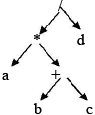2) syntax tree for if a=b then a:=c+d else b:=c-d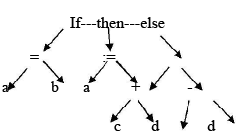e

• In three-address code, there is at most one operator on the right side of aninstruction; that is, no built-up arithmetic expressions are permitted.
x+y*z t1 = y * z t2 = x + t1

• Example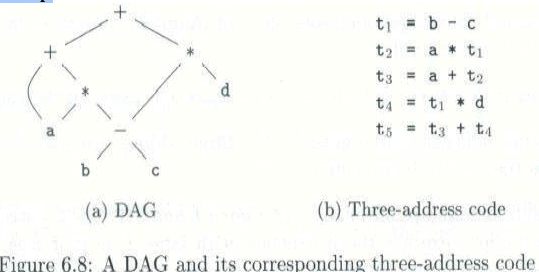Problems:
Write the 3-address code for the following expression
1. if(x + y * z > x * y +z) a=0;
2. (2 + a * (b – c / d)) / e
3. A :=b * -c + b * -c

• Example Three-address code is built from two concepts: addresses and instructions.
• An address can be one of the following:
– A name: A source name is replaced by a pointer to its symbol table entry.
• A name: For convenience, allow source-program names to Appear as addresses in three-address code. In an Implementation, a source name is replaced by a pointer to its symbol-table entry, where all information about the name is kept.
– A constant
• A constant: In practice, a compiler must deal with many different types of constants and variables
– A compiler-generated temporary
• A compiler-generated temporary. It is useful, especially in optimizing compilers, to create a distinct name each time a temporary is needed. These temporaries can be combined, if possible, when registers are allocated to variables.
A list of common three-address instruction forms: Assignment statements
– x= y op z, where op is a binary operation
– x= op y, where op is a unary operation
– Copy statement: x=y
–Indexed assignments: x=y[i] and x[i]=y
– Pointer assignments: x=&y, *x=y and x=*y
Control flow statements
– Unconditional jump: goto L
– Conditional jump: if x relop y goto L ; if x goto L; if False x goto L
– Procedure calls: call procedure p with n parameters and return y, is Optional param x1 param x2 …
param xn call p, n
– do i = i +1; while (a[i]<v);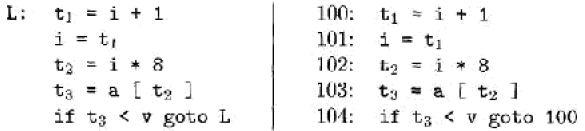The multiplication i * 8 is appropriate for an array of elements that each take 8 units of space.

• Three-address instructions can be implemented as objects or as record with fields for the operator and operands.
• Three such representations – Quadruple, triples, and indirect triples
• A quadruple (or quad) has four fields: op, arg1, arg2, and result.

Example D. Triples
• A triple has only three fields: op, arg1, and arg2
• Using triples, we refer to the result of an operation x op y by its position, rather by an explicit temporary name.

Example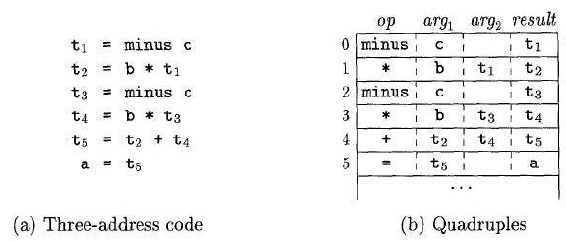d. Triples: • A triple has only three fields: op, arg1, and arg2
• Using triples, we refer to the result of an operation x op y by its position, rather by an explicit temporary name.

Example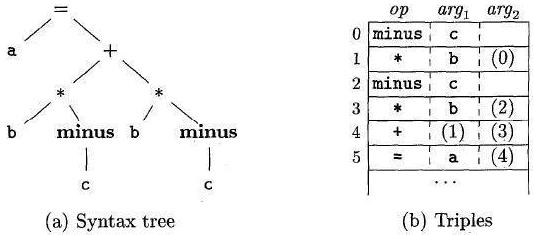Fig: Representations of a = b * - c + b * - c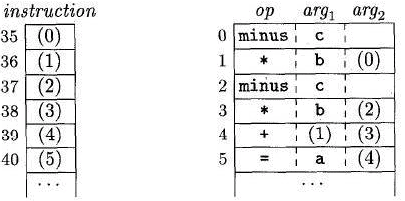Fig: Indirect triples representation of 3-address code
-> The benefit of Quadruples over Triples can be seen in an optimizing compiler, where instructions are often moved around.
->With quadruples, if we move an instruction that computes a temporary t, then the instructions that use t require no change. With triples, the result of an operation is referred to by its position, so moving an instruction may require changing all references to that result. This problem does not occur with indirect triples.

Single-Assignment

Static Form Static single assignment form (SSA) is an intermediate representation that facilitates certain code optimization.
• Two distinct aspects distinguish SSA from three –address code.

– All assignments in SSA are to variables with distinct names; hence the term static singleassignment.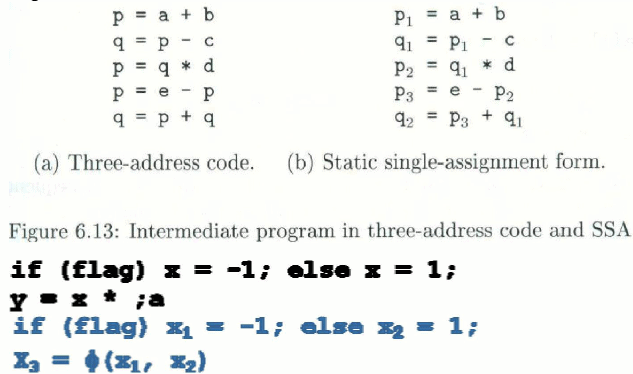2.  Type Checking:
•A compiler has to do semantic checks in addition to syntactic checks.

•Semantic Checks –Static –done during compilation –Dynamic –done during run-time

•Type checking is one of these static checking operations.

–we may not do all type checking at compile-time.

–Some systems also use dynamic type checking too.

•A type system is a collection of rules for assigning type expressions to the parts of a program.

•A type checker implements a type system.

•A sound type system eliminates run-time type checking for type errors.

•A programming language is strongly-typed, if every program its compiler accepts will execute without type errors.

In practice, some of type checking operations is done at run-time (so, most of the programming languages are not strongly yped).

Offer running on EduRev: Apply code STAYHOME200 to get INR 200 off on our premium plan EduRev Infinity!

## Compiler Design

16 videos|44 docs|29 tests

,

,

,

,

,

,

,

,

,

,

,

,

,

,

,

,

,

,

,

,

,

;